# Numerical Optimization of Drying Energy Consumption from Multiple Jets Impinging on a Moving Curved Surface

Numerical Optimization of Drying Energy Consumption from Multiple Jets Impinging on a Moving Curved Surface

Ali ChitsazanGeorg Klepp Birgit Glasmacher Kamyar Mohammad Pour

Institute for Energy Research, Ostwestfalen-Lippe University of Applied Sciences and Arts, Lemgo 32657, Germany

Institute for Multiphase Processes (IMP), Leibniz University Hannover, Hannover 30167, Germany

Institute of Fluid Dynamics and Thermodynamics, Otto von Guericke University Magdeburg, Magdeburg 39106, Germany

Corresponding Author Email:
ali.chitsazan@yahoo.com
Page:
755-762
|
DOI:
https://doi.org/10.18280/ijht.390309
8 July 2020
|
Accepted:
18 March 2021
|
Published:
30 June 2021
| Citation

OPEN ACCESS

Abstract:

Due to the increasing energy cost, the efficiency of the industrial dryer as the energy-intensive processes should be improved. The designer should optimize the design parameters of industrial drying equipment to achieve the minimum drying energy consumption. SST k-ω turbulence model is used to simulate a real geometry for industrial drying applications. For the optimization of the impinging round jet, the specific drying energy consumption is set as the objective function to be minimized. The jet to surface distance, jet to jet spacing, jet inlet velocity, jet angle, and surface velocity are chosen as the design parameters. The SHERPA search algorithm is used to search for the optimal point from the weighted sum of all objectives method. One correlation is developed and validated for the specific drying energy consumption. It is found that the SST k-ω turbulence model succeeded with reasonable accuracy in reproducing the experimental results. The minimum specific energy consumption correlates with high values of the jet to jet spacing, jet angle, and surface velocity and low values of the nozzle to surface distance and jet inlet velocity. The agreement in the prediction of the specific drying energy consumption between the numerical simulation and correlation is found to be reasonable and all the data points deviate from the correlation by less than 7%.

Keywords:

multiple jets, drying energy consumption, surface motion, surface curvature, CFD, optimization

1. Introduction

Jet impingements enhance the heat transfer rate in many industrial applications such as cooling, heating, and drying due to the large amounts of heat and mass transfer between the target surface and the working fluid. Jet impingement flow has many applications in the industry such as the drying of textile and paper, cooling of electronic and turbine components, etc. The designer should optimize the design parameters of industrial drying machines to achieve the minimum capital (size of the apparatus) and running (energy consumption) costs. Designs that require high jet velocity and temperature aren’t attractive due to the high energy costs .

Thermal drying is highly energy-intensive and competes with distillation as the most energy-intensive unit operation due to the high latent heat of water vaporization and the inherent inefficiency of using hot air as the most common drying medium [2, 3]. The national energy consumption for industrial drying operations ranging from 10-25% for developed countries . The thermal efficiency of industrial dryers varies from 30-60% which opens the opportunity for increasing efficiency . A typical convective dryer consumes five times its capital cost in energy in its lifetime and most of this energy is wasted. To make the dryer thermally more efficient, it is necessary to make innovative changes in the dryer design itself, reduce air leaks, and improve dryer insulation . Bahu et al.  have proven that proper insulation of the drying system and reducing air leakages can result in 26% reduction in energy consumption. Kudra et al.  have developed a simple excel based tool for the analysis of the energy performance of convective dryers.

Can et al.  determined that the maximum average Nu number for circular holes can be achieved at the optimum free area factor of about 0.03. Brevet et al.  identified an optimum H/d=2-5 and optimum S/d=4-5 to achieve the maximum heat transfer rates on a flat surface. Kamal et al.  stated that the uniform pressure distributions beside the largest drying rates can be satisfied at S/d = 3.5, H/d=6, and θ = 60°. Heo et al.  found that the optimum inclination angle and the pitch of staggered jet nozzles on a concave surface at a Re number of 23,000 are 59.09º and 8.074, respectively. Badra et al.  found that the optimal value of H/d=6-7 for the maximum stagnation Nu number from the single impinging jet. Xing et al.  found that H/d=3 could improve the heat transfer performance for a variety of crossflow configurations. Specht  found that for single nozzle arrays and hole channels the maximum heat transfer is at optimum S/d=6. For perforated plates, the maximum heat transfer is for S/d=4 and the minimum specific energy consumption is provided at S/d=8-10. Attalla  shows that the maximum average Nu achieved at S/d=2. Zhu et al.  found a relationship for uniform heat transfer (H≈8S). Bu et al.  determined an optimal H/d=4.5 to improve the heat transfer performance on a concave surface. Bu et al.  determined an optimal H/d=4-5.75 for the maximum stagnation Nu on a concave surface. Yang et al.  indicated that optimum values of H/d=10, S/d=30, and θ=15º enhance both local and averaged Nu on the concave surface.

The heat and mass transfer between multiple jets and a moving curved surface is more difficult to study due to the changing boundaries and effect of surface curvature but is also very relevant in engineering applications such as paper drying machines. Nevertheless, the effect of multiple jets impinging on the moving curved surface is omitted in the literature, and the focus is on the multiple round jets impinging on either the fixed curved surface or moving flat surface. A considerable amount of studies has been dedicated to the optimization of heat transfer in systems of multiple impinging jets only at optimum values for jet to surface distance, jet to jet spacing, and jet angle. While an optimum value of other key controlling design variables such as jet velocity and surface velocity is not observed in the literature. On the other hand, the optimization of the drying energy consumption in systems of multiple impinging jets on a moving curved surface is very rare in literature. Therefore, innovative changes in the dryer design are necessary to make the dryer thermally more efficient.

The scope of this research is to find the optimum value of key design parameters of paper drying machines such as the jet to surface distance, jet to jet spacing, jet inlet velocity, jet angle, and surface velocity. The specific drying energy consumption from multiple jets impinging on a moving curved surface is set as the objective function to be minimized. The weighted sum of all objectives method and the SHERPA search algorithm is used for the optimization study. In an optimization study, the objective is evaluated with respect to the design variables using a CFD model. The CFD model is based on the solution of the stationary Reynolds-averaged Navier-Stokes equation with a finite volume method. Finally, the optimum values are compared with the literature and one correlation is developed and validated for the specific drying energy consumption.

2. Definition of Characteristic Numbers

The specific drying energy is the energy for heating the air jet $\dot{H}_{j}$ related to the enthalpy of evaporation of the water $\dot{H}_{V}$:

$\frac{\dot{\mathrm{H}}_{\mathrm{j}}}{\dot{\mathrm{H}}_{\mathrm{v}}}=\frac{\pi \mathrm{d}^{2} \rho \mathrm{c}_{\mathrm{p}} \mathrm{V}}{4 \mathrm{~S}^{2}}\left(\frac{1}{\mathrm{~h}}+\frac{\mathrm{T}_{\mathrm{s}}-\mathrm{T}_{\mathrm{a}}}{\dot{\mathrm{m}}_{\mathrm{v}} \Delta \mathrm{h}_{\mathrm{V}}}\right)$    (1)

In the above equation, h is the local heat transfer coefficient, Ta the temperature of the ambient, cp the average specific heat capacity between the temperatures of jet and the environment, ρ the jet density, V the discharge velocity from the nozzles with a diameter of d, Ts saturation temperature, S nozzle pitch, $\dot{m}_{V}$ the evaporation flux, and ΔhV the evaporation enthalpy. The air jet temperature is calculated from the condition that the transferred heat has to cover the evaporation enthalpy and the enthalpy to heat the dry material flow from the ambient temperature (Ta) to the saturation temperature (Ts) .

The local heat transfer coefficient (h) is nondimensionalized to the Nusselt number by the following expression

$\mathrm{Nu}=\frac{\mathrm{hd}}{\mathrm{k}_{\mathrm{t}}}=\frac{\mathrm{q}}{\left(\mathrm{T}_{\mathrm{w}}-\mathrm{T}_{\mathrm{j}}\right)} \cdot \frac{\mathrm{d}}{\mathrm{k}_{\mathrm{t}}}$    (2)

where, q is the convective heat flux, Tw is the target wall temperature, Tj is the jet exit temperature, d is the jet exit diameter, ktis the thermal conductivity of the air at jet exit temperature and h is heat transfer coefficient.

3. Domain and Boundary Condition

Figure 1 shows the geometry of the impinging jet and boundary condition used in the present work. All jet inlets were modeled as circular planes in the top wall. The incoming flow assumed to be with constant fluid properties at T=298.15ºK, entered with a uniform velocity profile. The value of inlet velocity is so selected that it matches with the Re number over the range of 4337-21685. The target surface i.e. a moving curved surface was modeled as a no-slip wall held at a constant temperature Tw=60℃. On all other solid surfaces, no-slip with adiabatic wall boundary condition is imposed. Constant pressure outlet boundary condition is applied to all open boundaries. The movement of the curved surface is considered along curvilinear axes. A symmetric boundary condition was also applied in the X-Y plane for the central jet to reduce the computational cost [11, 12, 20].

## 1.png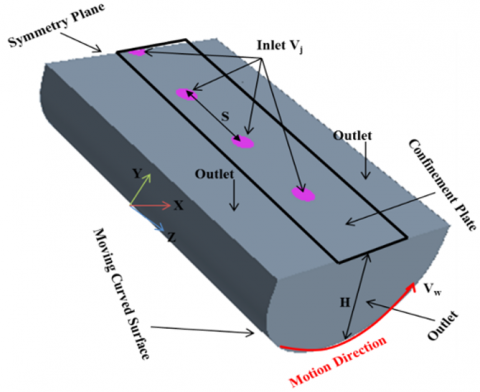Figure 1. Schematic of the computational domain

4. Computational Details

The numerical model is based on the solution of the stationary Reynolds-averaged Navier-Stokes equation with a finite volume method. The CFD model is set up and run with the commercial code STAR-CCM+ 13.02.013 by CD-Adapco. The final solution was obtained by applying a second-order discretization upwind scheme for the pressure, momentum, and energy terms, and the SIMPLE algorithm is used for pressure-velocity coupling and a segregated flow solver was used for all the calculations. SST k-ω turbulence model is used because it is recommended as the best compromise between computational cost and accuracy [11, 12]. The flow in the near-wall regime was simulated using a low-Reynolds number approach. The solution was considered to be converged when the value of the scaled residual of the continuity, momentum, and energy equations is less than 10-4.

5. Grid Generation and Sensitivity

An unstructured Polyhedral grid was generated using STAR-CCM+ auto-mesher. Boundary layers with a y+ value less than one are built on the target surface of the model.  The grid was refined near the curved target wall to enable better resolution of the flow in this part. The final numerical model accounted for about 2,157,431 grid cells.

A grid sensitivity study is carried out to ascertain the accuracy of the numerical results. It is carried out by analyzing the variation of Nu number distribution on the target surface along the Z-centerline (the lines pass through the stagnation points of jets). Three simulations with the same geometry but different grid sizes were carried out to check the grid independence of the simulation. The grid sizes are summarized in Table 1.

The local discretization error distribution is calculated by applying the GCI method . The overall discretization error for fine and intermediate grids was very small 2.6% and 4.12% respectively. Therefore, in the present case, the solution was grid-independent. To reduce the computational cost, the intermediate grid is selected as the final grid.

Table 1. Grid parameters of the refinement study at Re =23,000

 Grid Base Size(m) Cell Number Max y+ Average GCI % Course 0.00192 447431 0.44 --- Intermediate 0.00127 970045 0.31 4.21 Fine 0.00088 2157431 0.23 2.6
6. Design Variables and Objective Functions

In an optimization study, the objectives are evaluated with respect to the design variables using a CFD model. During the analysis, an optimization algorithm uses an embedded strategy to choose the values for the input parameters to best meet the analysis objectives.

Table 2. Design variables and design space

 Design variable Lower bound Upper bound H/d 2 10 S/d 2 10 θ 40 deg 90 deg Re number 4337 21685 Relative surface velocity (VR) 0.0034 1 Inlet velocity 10 m/s 50 m/s Surface velocity 0.17 m/s 10 m/s

The designer should optimize the design parameters of industrial drying equipment to achieve minimum energy consumption. Therefore, in the present optimization study, the specific drying energy consumption is selected as the objective function to be minimized. Optimization for the multiple jets impinging on a moving curved surface has been performed with respect to five key controlling design parameters as the jet to surface distance, the spacing between the jets, jet inlet velocity, surface velocity, and jet angle. These parameters can play a critical role in the rate of drying energy consumption and therefore optimization is required for design purposes. Other parameters, such as relative surface curvature (Cr=0.1), nozzle diameter (d=10mm), jet temperature (100℃), surface temperature (60℃), and ambient temperature (20℃) are held constant. The ranges of the design variables for the optimization have been concentrated on the paper drying machine as shown in Table 2.

7. Optimization Method

The weighted sum of all objectives is used as a numerical optimization method for the specific drying energy consumption on the moving curved surface to be minimized. The SHERPA search algorithm is used when running an optimization study. If the number of design variables (m) is less than 10, STAR-CCM+ recommended choosing the minimum design number of runs (N) equal to 10×m to make good progress. Therefore, in the present optimization study, minimum N should be considered equal to 50. To increase the accuracy of results, N is considered equal to 100 .

7.1 Weighted sum of all objectives

This type of optimization study allows an optimization based on a single objective or based on multiple objectives. For the multiple objectives, a linear weighting is used that combines all objectives into a single performance function. Running a weighted sum of all objectives analysis with multiple objectives returns a single best design. A design performance is based on the value returned for the objectives together with the degree to which a design satisfies its constraints. Once the constraints are satisfied, only the objectives contribute to the evaluation of performance using the following equation (see Figure 2):

$\sum_{i=1}^{N_{o b j}} \frac{\operatorname{Lin} W_{i} . \operatorname{Sign}_{i} . \mathrm{Obj}_{i}}{\mathrm{Norm}_{i}}-\sum_{j=1}^{N_{c o n}} \frac{\text { QuadWt }_{j} . \text { ConViol }_{j}^{2}}{\operatorname{Norm}_{j}^{2}}$    (3)

where:

• Nobj is the number of objectives in the design study.
• LinWti is the linear weight for the i-th objective.
• Signi is the sign for the i-th objective. The value is -1 for objectives being minimized and +1 for objectives being maximized.
• Obji is the response value for the i-th objective for that design.
• Normi is the normalization value for the i-th objective.
• Ncon is the number of constraints in the design study.
• QuadWtj is the quadratic weight for the j-th constraint. This value is 10000.0.
• ConViolj is the amount by which the j-th constraint is violated. ConViolj is 0.0 if the constraint is met.
• Normj is the normalization value for the j-th constraint, which is the value of the constraint itself. If the constraint value is 0, then the normalization value is 1.

The weighted sum of all objectives optimization uses the SHERPA search algorithm .

## 2.png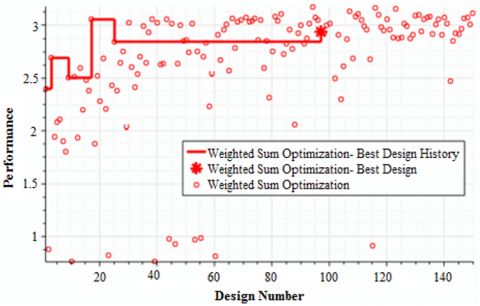Figure 2. Weighted sum of all objectives 

7.2 SHERPA algorithm

SHERPA Algorithm employs multiple search methods simultaneously rather than sequentially. This approach uses the best attributes of each search method. If a particular search method is deemed to be ineffective, this algorithm reduces its participation. While running this algorithm, a combination of global and local search methods is used. At any given time, the number of different methods that are used can range between two and ten. Unlike traditional optimization algorithms that require you to tune parameters manually, the tuning parameters in each method that SHERPA uses are modified automatically during the search. As it learns more about the design space, it determines when and to what extent to use each search method. Therefore, the SHERPA algorithm (Simultaneous Hybrid Exploration that is Robust, Progressive, and Adaptive); a combination of different search methods are used to optimize the efficiency. A comparison between a traditional optimization approach and this approach is displayed below (see Figure 3) .

## 3.png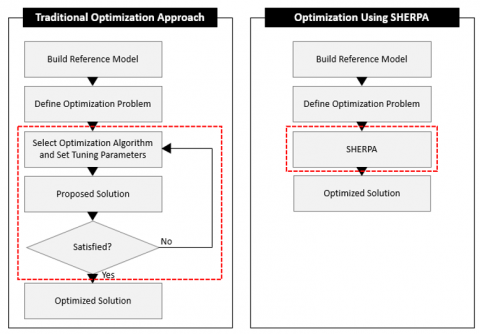Figure 3. A comparison of a traditional optimization approach and the SHERPA approach 

8. Results and Discussion

8.1 Evaluation of computational model

For numerical predictions of multi-jet impingement heat transfer, a quantification of the numerical accuracy is equally significant. Figure 4 indicates the local Nu distributions along the curvilinear axis on the target surface. The CFD results of this work have been compared with the available data of Fenot et al.  who investigated the heat transfer due to a row of air jets impinging on a fixed concave surface. Uniform heat flux of 4000 W/m2 was applied to the target surfaces to simulate the condition of the target wall in the experiment. The difference between the experiment and the corresponding values of CFD data is approximately 15% on average. The agreement between the two is very good and closely followed the same trend as the experimental data. It can be concluded from the evaluation of the turbulence model concerning the predictions of heat transfer that the computational model (discretization, numerical scheme, turbulence model) represents a good compromise between the accuracy of its results and the computational effort.

## 4.pngFigure 4. Comparison of the local Nu distributions along curvilinear axis on fixed curved surface from experiments and CFD (H/d=5, S/d=4, Re = 23000, Cr=0.1, VR=0)

8.2 Optimization results

8.2.1 Jet Re number

Figure 5 shows the design study with a logarithmic trend line during the numerical optimization for the specific drying energy consumption versus the jet Re number.

## 5.png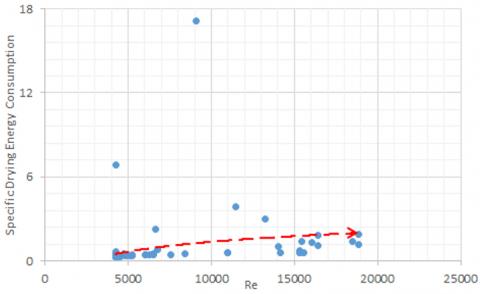Figure 5. Design study during the numerical optimization for the specific energy consumption versus Re

Increasing the Re number increases the specific energy consumption. Because increasing the Re number occurs with increasing the jet inlet velocity. Therefore, the minimum specific energy consumption is expected to occur for minimum inlet velocity due to the role of inlet jet velocity in the numerator of specific energy consumption definition (see Eq. 1))).

8.2.2 Nozzle to surface distance

Figure 6 shows the design study with a logarithmic trend line during the numerical optimization for the specific drying energy consumption versus nozzle to surface distance (H/d).

## 6.png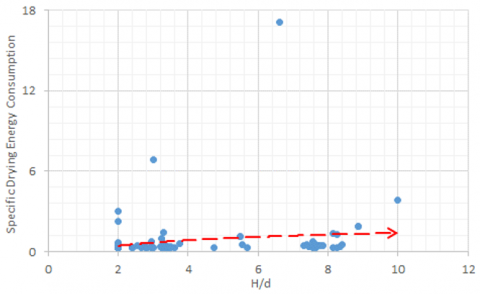Figure 6. Design study during the numerical optimization for the specific energy consumption versus H/d

The specific energy consumption increases as H/d increases due to the decrease in the average heat transfer coefficient (see Figure 7). Therefore, the minimum specific energy consumption is expected to occur at a minimum H/d value (see Figure 6).

## 7.png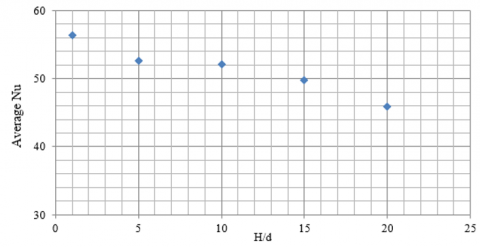Figure 7. Effects of the nozzle to surface distance on the total average Nusselt number on the moving curved surface (Re=23000, S/d=4, and VR=0.28)

## 8.png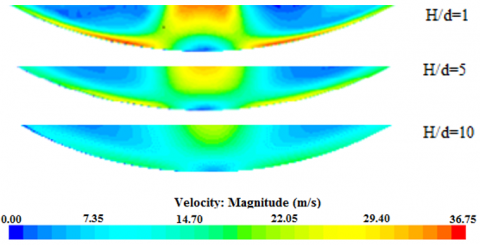Figure 8. Effects of the nozzle to surface distance on the velocity magnitude (Re=23000, S/d=4, VR=0.28)

The heat transfer coefficient increases as H/d decreases. Increasing H/d, the momentum exchange between the jet flow and the ambient increases, hence the flow in case of low H/d value has more momentum compared to the higher H/d values leads to the higher average and local heat transfer coefficient (see Figures 7 and 8).

8.2.3 Jet to jet spacing

Figure 9 shows the design study with a logarithmic trend line during the numerical optimization for the specific drying energy consumption versus jet to jet spacing (S/d).

## 9.png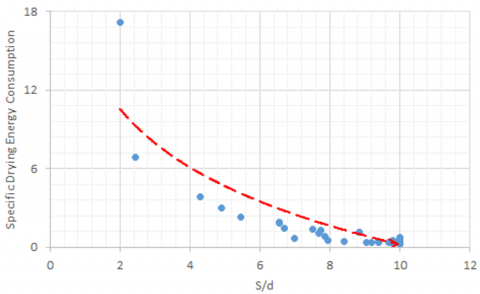Figure 9. Design study during the numerical optimization for the specific energy consumption versus S/d

The specific energy consumption is highly dependent on the jet to jet spacing and it decreases with increasing S/d due to the role of the jet to jet spacing in the denominator of specific energy consumption definition (see Eq. (1)) and also increasing the jet to jet spacing increases the average heat transfer coefficient (see Figure 10). Therefore, the minimum specific energy consumption is expected to occur at the maximum S/d value (see Figure 9).

## 10.png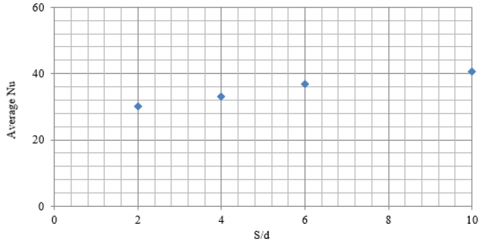Figure 10. Effects of the jet to jet spacing on the total average Nusselt number on the moving curved surface (Re=23000, H/d=2, VR=0.28)

## 11.png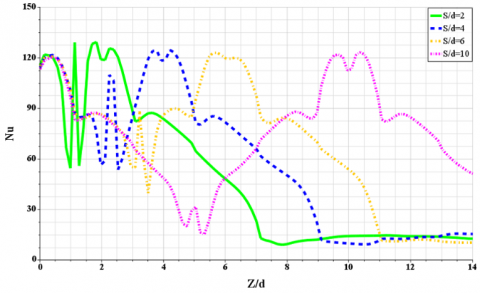Figure 11. Effects of the jet to jet spacing on the local Nu along Z-axis (Re=23000, H/d=2, and VR=0.28)

Increasing the jet to jet spacing increases the average heat transfer coefficient due to the lower jet interaction. Because the wall flows of the two jets are impinging upon each other and forming a new stagnation region. This affects the local distribution of the heat transfer coefficient (see Figures 10 and 11).

8.2.4 Jet angle

Figure 12 shows the design study with a logarithmic trend line during the numerical optimization for the specific drying energy consumption versus jet angle (θ). The jet angle is varied between 40° and 90° as measured with respect to the horizontal axes. The case of 90° corresponds to the orthogonal jet and jet angles less than 90° correspond to the angled jets.

## 12.pngFigure 12. Design study during the numerical optimization for the specific energy consumption versus θ

The specific energy consumption is relatively insensitive to the jet angle in contrast to the heat transfer coefficient. When the jet impinging orthogonally on the surface (90°); it can pick up the most heat upon striking the surface. Hence, the surface average Nu number increases with increasing the jet angle and the maximum average Nu number is expected to occur for orthogonal jets (see Figure 13).

## 13.png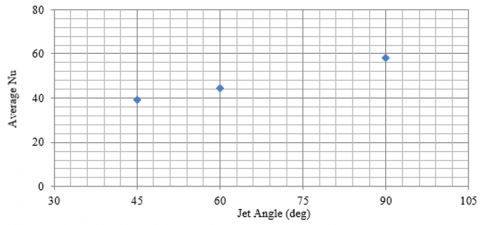Figure 13. Effects of jet angle on the total average Nusselt number on the moving curved surface (Re=23000, H/d=1, S/d=4, and VR=0.28)

8.2.5 Relative surface velocity

Figure 14 shows the design study with a logarithmic trend line during the numerical optimization for the specific drying energy consumption versus relative surface velocity (VR). The specific energy consumption decreases with increasing the relative surface velocity due to a lower time constant associated with the heat and mass transfer rate to or from the target surface. Therefore, the minimum specific energy consumption is expected to occur at maximum relative surface velocity.

## 14.png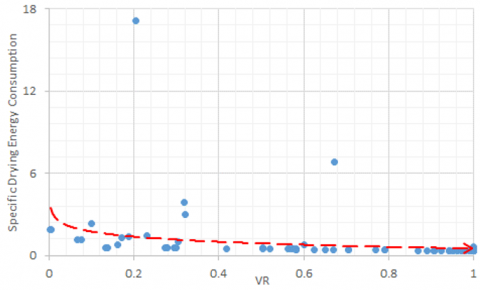Figure 14. Design study during the numerical optimization for the specific energy consumption versus VR

Table 3 shows a summary of results during the numerical optimization. It can be concluded that the minimum specific energy consumption correlates with high values of the jet to jet spacing (S/d), jet angle (θ), and surface velocity (Vw) and low values of the nozzle to surface distance (H/d) and jet exit velocity (Vj). Therefore, the best design study is found at H/d=3.3, S/d=10, θ=88°, Vj=10m/s and Vw=10 m/s for the investigated parameters in the range of H/d=2-10, S/d=2-10, θ=40-90°, Vj=10-50 m/s and Vw=0.17-10 m/s under d = 10 mm as shown in Table 3. Generally, the best designs are in the range of H/d= 3.3-3.6, S/d= 10, θ= 88-90°, Vj = 10 m/s and Vw =10m/s. These results have a correlation with the findings of Specht  who state that the minimum specific drying energy consumption is achieved with slower jet exit velocities, the distance between the nozzle and surface (H/d) above four diameters, and jet to jet spacing (S/d) between 8 and 10.

Table 3. Results during the numerical optimization

 Objectives H/d S/d θ (deg) Vj (m/s) Vw (m/s) Min SDEC 3.3 10 88 10 10
9. Correlation Equation

One correlation for the specific drying energy consumption (SDEC) is developed for the single row of jets impinging on a moving curved surface. A multiple regression fit is applied for the development of correlation from the numerical simulation (hundred design points during the numerical optimization):

$\mathrm{SDEC}=0.35 \mathrm{Re}^{0.58}(\mathrm{H} / \mathrm{d})^{0.034}(\mathrm{~S} / \mathrm{d})^{-2.2} \theta_{\mathrm{rad}}^{-0.003}(\mathrm{VR})^{-0.018}$    (4)

The above correlation is proposed in terms of Re, H/d, S/d, θ, and VR as the independent variables for Re number in the range of 4337 to 21685, H/d from 2 to 10, S/d from 2 to 10, θ from 40 to 90° and VR from 0.0034 to 1. The observed trends in numerical simulation and optimization are shown by the exponents of the independent parameters in the correlation. The agreement in the prediction of the specific drying energy consumption between the numerical simulation and correlation is found to be reasonable and all the data points deviate from the correlation by less than 7% (see Figure 15).

## 15.png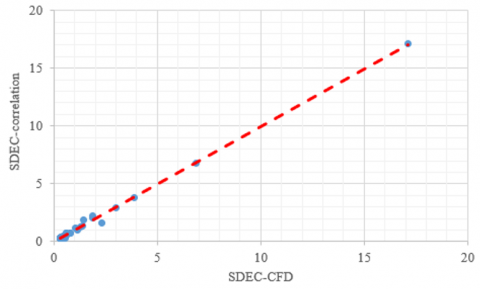Figure 15. Parity plot showing a comparison between the specific drying energy consumption predicted by the CFD and correlation

10. Conclusion

The numerical simulations and optimization of multiple circular jets impinging on a moving curved surface are carried out and the commercial CFD package STAR CCM+ is employed with the SST k-ω turbulence model to simulate a real geometry for industrial drying applications. The designer should optimize the design parameters of industrial drying equipment to achieve minimum energy consumption. The optimization has been performed with respect to five design parameters as the jet to surface distance, the spacing between the jets, jet inlet velocity, surface velocity, and jet angle. For the optimization of the impinging jet, the specific drying energy consumption on the moving curved surface is set as the objective function to be minimized. Hundred design points are selected by the SHERPA search algorithm within the design space. One correlation describing the specific drying energy consumption has been developed. The agreement in the prediction of the specific drying energy consumption between the numerical simulation and correlation is found to be reasonable. The results of this research lead to effective heat and mass transfer processes or a significant reduction in drying energy consumption for industrial drying applications.

Nomenclature
 Af open area ratio, total jet area to heat transfer area cp specific heat capacity (J/kg K) ConViolj violated amount by j-th constraint d jet exit diameter (m) h heat transfer coefficient (W/m2K) H nozzle to surface distance (m) kt thermal conductivity (W/mK) LinWti linear weight for i-th objective m number of design variables N number of runs Ncon number of constraints Nobj number of objectives Normi normalization value for i-th objective Normi normalization value for i-th objective Normj normalization value for j-th constraint Nu Nusselt number Obji i-th objective q convective heat flux (W/m2) QuadWtj quadratic weight for j-th constraint Re Reynolds number S jet to jet spacing (m) Signi sign for i-th objective T temperature (K) V jet exit velocity (m/s) X, Y, Z coordinates y+ dimensionless wall distance ΔhV evaporation enthalpy (J/kg) $\dot{\mathrm{m}}_{\mathrm{v}}$ evaporation flux (Kg/m2s) $\dot{\mathrm{H}}_{\mathrm{v}}$ enthalpy of water evaporation (J/s) $\dot{\mathrm{H}}_{\mathrm{j}}$ energy for heating the air jet (J/s) Greek letters k turbulence kinetic energy (m2s-2) ω specific dissipation rate of turbulence kinetic energy (1/s) θ jet angle (deg) ρ density (kg/m3) Subscripts a ambient ave average j jet opt optimum s saturation w wall Abbreviation Cr curvature ratio; nozzle to surface diameter CFD computational fluid dynamic GCI grid convergence index VR velocity ratio; surface to jet velocity SDEC specific drying energy consumption SST shear stress transport
References

 Etemoglu, A.K., Can, M. (2013). Performance studies of energy consumption for single and multiple nozzle systems under impinging air jets. J. Heat Mass Transfer, 49: 1057-1070. https://doi.org/10.1007/s00231-013-1137-8

 Mujumdar, A.S. (2006). Handbook of Industrial Drying, 3rd Ed; CRC Press: Boca Raton, FL.

 Mujumdar, A.S. (2008). Guide to Industrial Drying: Principles, Equipment and New Developments, 3rd Ed. Three S Colors Publications, India.

 Gong, Z.X., Mujumdar, A.S. (2008). Software for design and analysis of drying systems. J. Drying Technology, 26: 884-894. https://doi.org/10.1080/07373930802142390

 Baker, C.G.J. (2005). Energy efficient dryer operation–an update on developments. J. Drying Technology, 23: 2071-2087. https://doi.org/10.1080/07373930500210556

 Bahu, R.E., Baker, C.G.J., Reay, D. (1983). Energy balances on industrial dryers-A route to fuel conservation. J. Separation Processes Technology, 4: 23-28.

 Kudra, T., Platon, R., Navarri, P. (2009). Excel-based tool to analyze the energy performance of convective dryers. J. Drying Technology, 27: 1302-1308. https://doi.org/10.1080/07373930903383687

 Can M., Etemoglu, A.B.E. (2002). A. Experimental study of convective heat transfer under arrays of impinging air jets from slots and circular holes. J. Heat Mass Transfer, 38: 251-259. https://doi.org/10.1007/s002310100249

 Brevet, P., Dejeu, C., Dorignac, E., Jolly, M., Vullierme, J. (2002). Heat transfer to a row of impinging jets in consideration of optimization. Int. J. Heat Mass Transfer, 45: 4191-4200. https://doi.org/10.1016/S0017-9310(02)00128-X

 Kamal, R.M., El sayed Mostafa, M., Abdel Aziz, S.S. (2006). An experimental study of an oblique multiple circular air jets impingement on a flat plate. Eight International Congress of Fluid Dynamics and Propulsion, Egypt.

 Heo, M.W., Lee, K.D., Kim, K.Y. (2012). Parametric study and optimization of staggered inclined impinging jets on a concave surface for heat transfer augmentation. J. Numerical Heat Transfer, Part A: Applications, 61: 442-462. https://doi.org/10.1080/10407782.2012.654453

 Badra, J., Masri, A., Behnia, M. (2013). Enhanced transient heat transfer from arrays of jets impinging on a moving plate. J. Heat Transfer Engineering, 34: 361-371. https://doi.org/10.1080/01457632.2013.717046

 Xing, Y., Weigand, B. (2013). Optimum jet-to-plate spacing of inline impingement heat transfer for different crossflow schemes. J. Heat Transfer, 135: 072201. https://doi.org/10.1115/1.4023562

 Specht, E. (2014). Impinging jet drying, modern drying technology, Volume 5: Process Intensification, First Edition. Published by Wiley-VCH Verlag GmbH & Co. KGaA.

 Attalla, M. (2015). Stagnation region heat transfer for circular jets impinging on a flat plate, experimental heat transfer. J. Thermal Energy Generation, Transport, Storage, and Conversion, 28: 139-155. https://doi.org/10.1080/08916152.2013.829134

 Zhu, D., Wang, Y., Zhu, J. (2015). Heat transfer characteristics of multinozzle air impingement jet during die steel plate cooling progress. J. Advances in Mechanical Engineering, 698072. https://doi.org/10.1155/2014/698072

 Bu, X., Peng, L., Lin, G. (2015). Experimental study of jet impingement heat transfer on a variable-curvature concave surface in a wing leading edge. J. Heat and Mass Transfer, 90: 92-101. https://doi.org/10.1016/j.ijheatmasstransfer.2015.06.028

 Bu, X., Peng, L., Lin, G. (2016). Jet impingement heat transfer on a concave surface in a wing leading edge: Experimental study and correlation development. J. Experimental Thermal and Fluid Science, 78: 199-207. https://doi.org/10.1016/j.expthermflusci.2016.06.006

 Yang, B., Chang, S., Wu, H., Zhao, Y., Leng, M. (2017). Experimental and numerical investigation of heat transfer in an array of impingement jets on a concave surface. J. Applied Thermal Engineering, 127: 473-483. https://doi.org/10.1016/j.applthermaleng.2017.07.190

 Fenot, M., Dorignac, E., Vullierme, J.J. (2008). An experimental study on hot round jets impinging a concave surface. J. Heat and Fluid Flow, 29: 4945-4956. https://doi.org/10.1016/j.ijheatfluidflow.2008.03.015

 Roache, P.J. (2003). Conservatism of the grid convergence index in finite volume computations on steady-state fluid flow and heat transfer. J. Fluids Engineering, 125: 731-735. https://doi.org/10.1115/1.1588692

 STAR-CCM+ 13.02.013 user guide by CD-Adapco. Siemens PLM. STAR-CCM+. https://www.plm.automation.siemens.com/, accessed on Jan. 15, 2018.